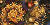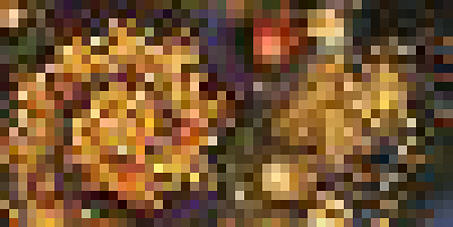Lesson 1     Lesson 2     Lesson 3     Lesson 4     Lesson 5     Lesson 6     Additional Information     Glossary of Terms     Links & Resources Lesson 1 – Page 2:How pixels are coloredFigure 2: Foisoun © 2005 Janet Parke     In Lesson 1 we're going to begin learning about the nature of fractals and exploring their amazing structure. There are many types of fractals. The three most common categories are: escape-time fractals – Mandelbrot, Lyapunov, Phoenix, Newton, etc.; Iterated Function Systems (called IFS fractals) – of which Flame fractals is a subset; and random fractals, which are generated by stochastic rather than deterministic processes. For the purposes of this course, we are going to focus entirely on escape-time fractals – the Mandelbrot and Julia structures, specifically. Once the basic concepts are learned using these fractal formulas, they can then be applied and adapted to explorations using other calculation formulas. Familiar fractal structures can then provide a benchmark for use in exploring the various coloring formulas. [Note: this lesson contains fractal-speak and mathematical terms that may be unfamiliar to you. Please check the Glossary (link above) for definitions and explanations. And be sure to let me know if there are terms I've missed or that are still unclear.]     It's important to have at least a rudimentary understanding of how a fractal image appears on your computer screen. Escape-time fractals are rendered with a special type of formula that takes a complex number (a number which has two parts – a real part, and an imaginary part) – performs a specified set of actions upon that number, and then produces a result. The result is then iterated (fed back into the formula repeatedly) until some pre-determined condition (called the bailout) is met. Once the bailout threshold is crossed and a numerical result has been determined, the coloring formula selected in Ultra Fractal uses that result to assign a color to the pixel.     So... calculating and rendering a Mandelbrot fractal on your computer screen works like this: 1. The variable z represents the number that will be fed into the fractal formula. It is initialized to the complex number 0/0. (0/0 is mathematical notation for a complex number that means that the real and imaginary parts of z are both set to 0, not that z equals 0 divided by 0.) The variable c represents, and is initialized to, the pixel's coordinates on the complex plane. 2. These variables (z and c) are then plugged into the Mandelbrot formula (z = z² + c). 3. After the first iteration of the formula, wherein z is squared and then added to the value of c, the resulting new value of z is evaluated against the bailout condition. The default bailout for the Mandelbrot set in Ultra Fractal (|z| <= 4) states that the magnitude of z (written |z|, which means the size of z) must be greater than 4 to bail out. If the magnitude of z is less than or equal to 4, then z will be fed back into the next iteration of the formula 4. If |z| crosses (is larger than) the bailout threshold, the pixel is an outside point and the value of z is passed to the outside coloring formula. There, it is assigned a number based on the gradient index and the pixel is colored the corresponding color. 5. If |z| does not exceed the bailout, z is repeatedly fed back into the formula. 6. If |z| doesn't cross the bailout threshold by the designated number of maximum iterations, the pixel is considered to be an inside point. The current value of z is then passed to the inside coloring formula and the pixel is colored accordingly. 7. Once the pixel is colored, Ultra Fractal moves to the next pixel and this process continues until all the pixels in the image have been calculated and colored.Figure 3 (at left) is a 50 pixel by 25 pixel rendering of the image at the top of the page. Each pixel is a tiny point of color on the screen – much too small to see individually.Figure 4 Figure 4 is a magnification of Figure 3, where each pixel is now expanded into a small block of color. For each pixel, the formula iterated until the bailout threshold was crossed, then the pixel was assigned the color you see in each block above. Every image is calculated and colored this way – one pixel at a time. Back – Lesson 1 – Page 3 Copyright © 2005-2011 Janet Parke